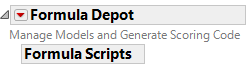Predictive and Specialized Modeling > Formula Depot > Launch the Formula Depot Platform
Publication date: 11/10/2021

#Launch the Formula Depot Platform

Launch the Formula Depot by selecting Analyze > Predictive Modeling > Formula Depot.

Figure 13.3 Empty Formula Depot from LaunchAlternatively, if there is not an open Formula Depot then a Formula Depot opens when you select a Publish command.

##Platforms That Publish Prediction Formulas to the Formula Depot

The platforms that publish prediction formulas and generate scoring code include:

Discriminant

Least Squares Regression

Logistic Regression

Partition

Uplift

K Nearest Neighbors

Naive Bayes

Neural

Latent Class Analysis

Principal Components

Generalized Regression

PLS

Gaussian Process

In platforms that do not publish prediction formulas to the Formula Depot, you can save the prediction formula to the data table. From the data table, add it to the Formula Depot by selecting Add Formula from Column. However, the scoring code might not be fully functional for such models. For more information about scoring code see Generating Scoring Code from the Formula Depot Platform.

In addition, you can publish a formula directly from the formula editor. Select the formula, right-click, and select Publish expression to formula depot.